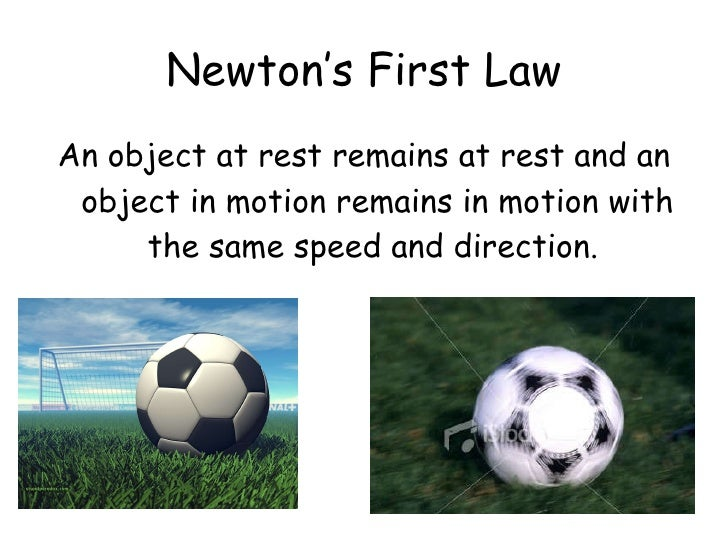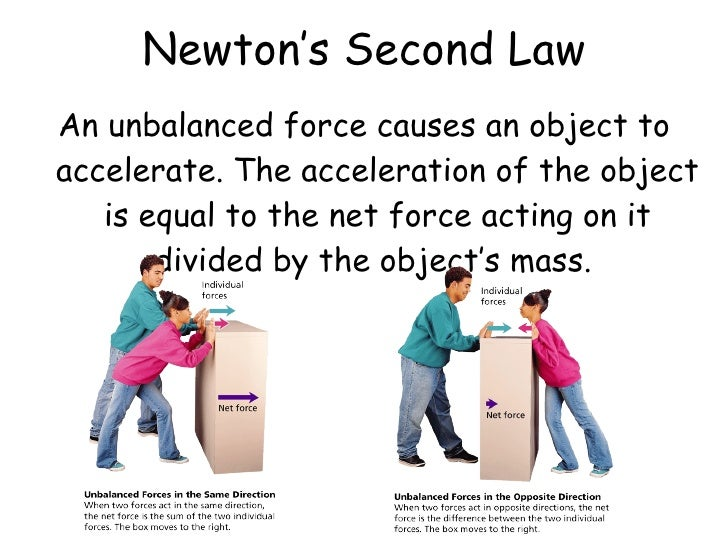# What is newton's law?

+1 vote
2.9k views
in Physics
retagged
What is newton's law?

by (65.0k points)
selected

1. First Law Of Motion:

Every object in a state of uniform motion tends to remain in that state of motion unless an external force is applied to it.

2. Second Law Of Motion:

The change of momentum of a body is proportional to the impulse impressed on the body, and happens along the straight line on which that impulse is impressed.
In simple terms, if the mass of the body is constant, it can be restated as :
"The acceleration of an object is directly proportional to the sum of all the forces acting on the object and inversely proportional to the mass"

3. Third Law Of Motion:

To every action there is always opposed an equal reactionNewton's Third Law Of Motion